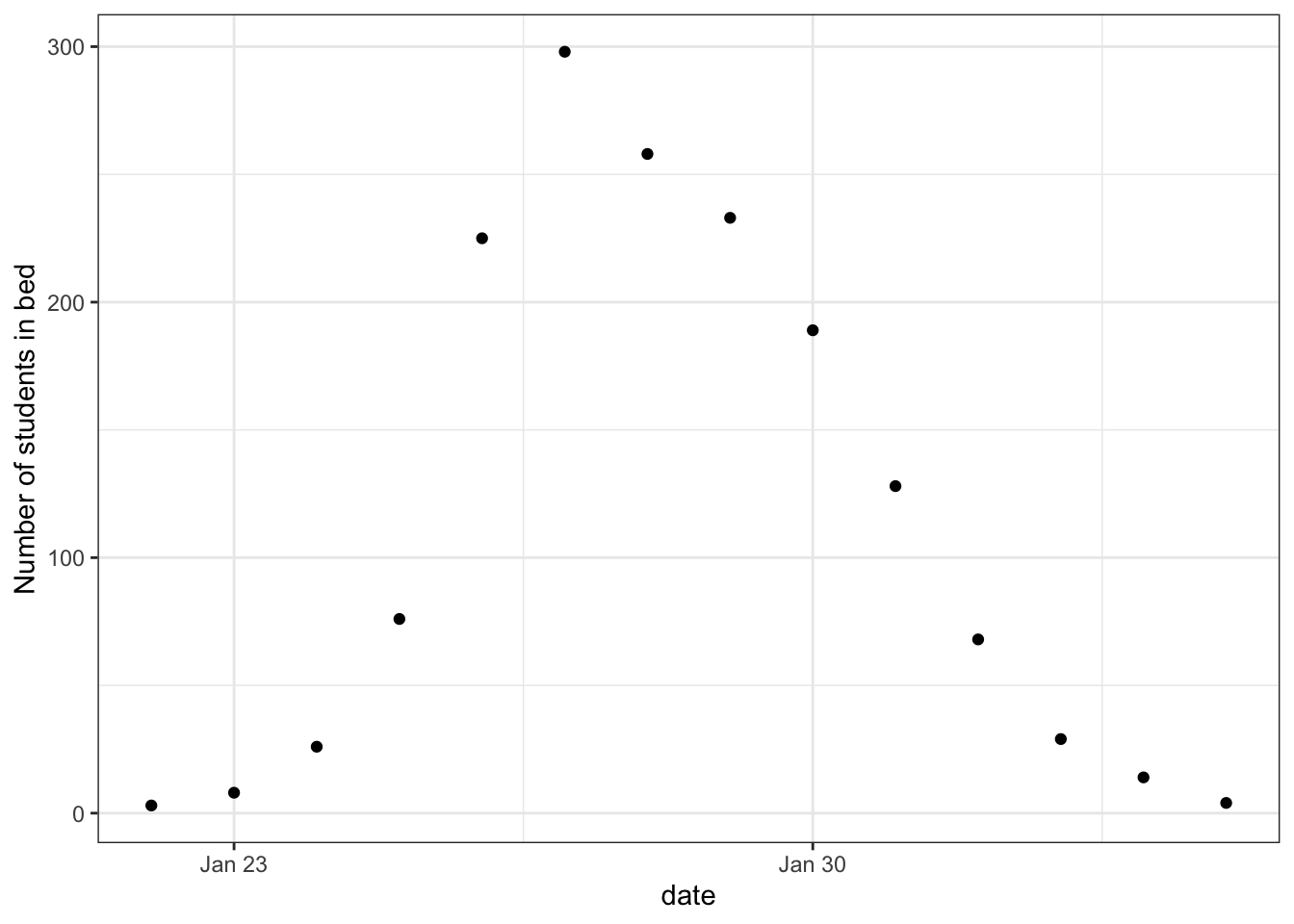# Introduction

The ongoing pandemic of the Coronavirus COVID-19 has led to an increased interest in statistical disease modeling and, amongst other approaches, Bayesian modeling (e.g Flaxman et al. (2020); Riou et al. (2020); see this post on the Stan forum for an updated list of examples).

Transmission models of infectious diseases can help answer questions about past and future transmission, including the effects of interventions. Population-based models subdivide the total population into homogeneous groups, called compartments. The flows between compartments can be described by a system of differential equations. Individuals within a compartment are considered to be in the same state (e.g. susceptible, infected, etc.). Moreover, the time-dependent volume of each compartment solves a system of ordinary differential equations (ODEs). Compartment models are relatively easy to formulate and computationally tractable.

In this tutorial, we demonstrate with a simple example how to formulate and fit a typical compartmental model in Stan. Stan is a probabilistic programming framework designed to let the user focus on modeling, while inference happens under the hood (Carpenter et al. 2017). This allows for faster implementation and extension of epidemiological models through a principled modeling workflow, and is thus particularly useful when handling rapidly evolving data and knowledge. Stan is an expressive language that supports many probability densities, matrix operations, and numerical ODE solvers. We can combine all these elements to specify a data generating process. We can also compute useful epidemiological parameters, such as the basic reproduction number, \(R_0\), and make predictions.

Generative models formulated in Stan can be used both for simulation and inference. Stan bolsters several inference methods: full Bayesian inference using Markov chains Monte Carlo (MCMC), approximate Bayesian inference with variational inference, and penalized maximum likelihood estimation. We focus on Bayesian inference with MCMC. Bayesian inference gives us a principled quantification of uncertainty and the ability to incorporate domain knowledge in the form of priors, while MCMC is a reliable and flexible algorithm. In addition, Stan provides diagnostic tools to evaluate both the inference (e.g. accuracy of the MCMC, convergence of chains) and the model (e.g. posterior predictive checks).

Other tutorials on the subject include the work by Chatzilena et al. (2019) and Mihaljevic (2016) on transmission models, and the case studies by Carpenter (2018), Weber (2018), and Margossian and Gillespie (2017a) on ODE-based models, all of which can serve as complementary reading.

## Outline

Through the lens of the Susceptible-Infected-Recovered (SIR) model, we show how to put together a principled Bayesian workflow in Stan, allowing a faster development of reliable epidemiological models. In Section 1, we introduce how to build, fit, and diagnose compartment models in Stan. The next sections discuss topics that can help practitioners fit more complex models. Section 2 reviews how we can use simulated data to examine our model and our priors, and provides an introduction to inference calibration. Section 3 offers a pragmatic discussion on how to efficiently implement and scale up ODEs in Stan. Throughout the tutorial, we use R as a scripting language5, and, while we review some elementary concepts, assume the reader has basic familiarity with Bayesian inference and Stan. The source code of this case study can be found on Github here.

# 1 Simple SIR

## Data

In this example, we examine an outbreak of influenza A (H1N1) in 1978 at a British boarding school. The data consists of the daily number of students in bed, spanning over a time interval of 14 days. There were 763 male students who were mostly full boarders and 512 of them became ill.  The outbreak lasted from the 22nd of January to the 4th of February. It is reported that one infected boy started the epidemic, which spread rapidly in the relatively closed community of the boarding school. The data are freely available in the R package outbreaks, maintained as part of the R Epidemics Consortium.

``````library(outbreaks)
library(tidyverse)``````
``head(influenza_england_1978_school)``
``````##         date in_bed convalescent
## 1 1978-01-22      3            0
## 2 1978-01-23      8            0
## 3 1978-01-24     26            0
## 4 1978-01-25     76            0
## 5 1978-01-26    225            9
## 6 1978-01-27    298           17``````
``````theme_set(theme_bw())
ggplot(data = influenza_england_1978_school) +
geom_point(mapping = aes(x = date, y = in_bed)) +
labs(y = "Number of students in bed")``````## Mathematical transmission model

The Susceptible-Infected-Recovered (SIR) model splits the population in three time-dependent compartments: the susceptible, the infected (and infectious), and the recovered (and not infectious) compartments. When a susceptible individual comes into contact with an infectious individual, the former can become infected for some time, and then recover and become immune. The dynamics can be summarized graphically: